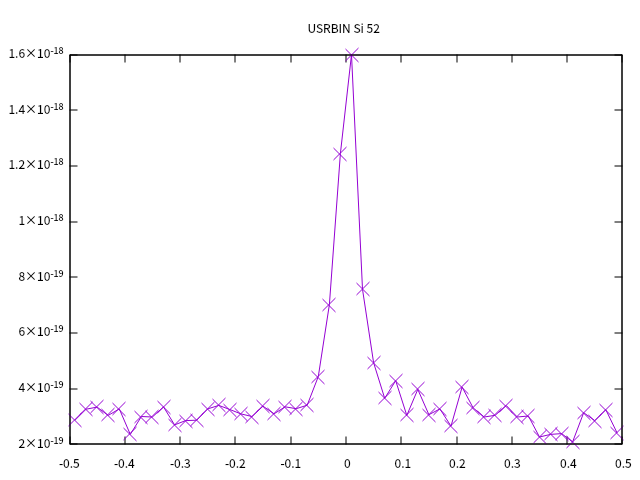# Overestimating dpa compared to the value in the literature

Hello,

I try to reproduce the maximum dpa calculated by this paper
2013 On thermal and radiation damage to silicon crystals in the LHC proton beam.pdf (1.3 MB)
. The maximum dpa calculated by the above paper is 0.4 dpa/cm^3/test.

The following is my calculations.
This is my input file
Si.inp (1.2 KB)
. Here is the distribution of maximum dpa along y direction from my calculationswhich is comparable with the top panel of fig. 1 in the above paper. However, the peak value calculated here is 1.6E-18 dpa ( 2.4E20 p/cm^2 * 0.24E-2 cm^2) / (202001001E-12 cm^3) = 2304000 dpa/cm^3/test, which is larger than 0.4 dpa/cm^3/test in the above paper.

Could someone help to have a look if there is any misunderstanding of the output of fluka or there is any error in my inputfile?

Well, the discrepancy between 2.3e6 and 0.4 is quite suspicious.
It comes from a misunderstanding related to the physical units of the concerned quantity, which are wrongly reported in the paper itself. In fact, talking about dpa/cm^3 makes no sense: we are dealing with Displacements Per Atom, given by the ratio between the number of displacements in the considered volume and the number of atoms in that volume. The scoring volume is already accounted for (by FLUKA too) in the calculation of the number of atoms it contains, so the (dimensionless) unit is just dpa. Thereby, you get 1.6e-18 dpa per incident proton to be multiplied by a proton population of 2.4e20 p/cm^2 (quoted proton fluence) times 0.24e-2 cm^2 (simulated uniform beam spot), so yielding 0.92 dpa.
The remaining factor of ~2 discrepancy is due to the fact that in the paper a Gaussian - rather than uniform - beam spot was simulated, with the same proton population as above. This, however, translates into a central proton fluence that is actually about one half of the quoted one, because the considered 0.24e-2 cm^2 area (corresponding to one sigma rectangle) contains only (less than) one half (0.68 times 0.68, which is the one sigma Gaussian integral) of the total proton population.
Therefore, for a proton fluence of 2.4e20 p/cm^2, the correct number is 0.9 dpa.

These explanations help to solve my confusion. Thank you very much.